ABC - Computer
ABC - Operator's Manual

Work in progress, just beginning, as of April 8, 2010

Overview of the ABC
Much of the following is a selection from that web site.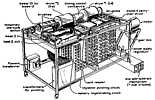Overview drawing from http://www.scl.ameslab.gov/Projects/ABC/Progress.html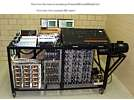Front view - from http://www.scl.ameslab.gov/Projects/ABC/Progress.html
 The following major tasks could be performed by one or more people, depending ... ABC Customer Interface / Mathematician - advise customers of capabilities of machine and suitable problems - select an initial scaling factor - prepare customer equations for keypunching - - possibly suggest equation sequencing for increased accuracy ... - schedule work on machine - attempt to verify machine results, possible machine or operator error - return results to customer, and consult ABC Machine Operator - perform regular test program to help assure ABC functioning correctly - accept input equations from Customer Interface - keypunch input equations - ? pre convert decimal card(s) data to a single binary "card" ? for each equation - - this step reduces operating complexity and confusion - perform the machine operations using Gaussian Elimination phases - - needs to determine the relative absolute values of pivot points - - possible aid of "binary column comparator", to be specified - - - (which binary card of pair to load onto the KA drum) - - if machine overflow, rescale the two inputs and try again. - convert the obtained single unknown equations to decimal, - - and record values onto output work sheets - using a desk calculator, and the single unknown equation on the work sheets - - determine the decimal value of each unknown - give results, and comments, to Customer Interface ABC Machine Technician - perform regular test program to help assure ABC functioning correctly - using technical expertise, repair any faults.
 Operator's Panel, The annotation overflows onto a second image Image from Charles Shorb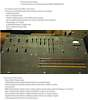Top Rows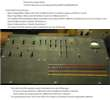Bottom Rows

KeyPunch

 The way, (or the only "reasonable" way?) to get starting data into the ABC is via punched "IBM Cards" Here is an inexpensive Key Punch of the era. (maybe an IBM Type 011 Electric Key punch?) Photo source unknown -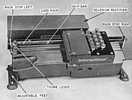Overview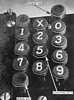Keyboard Detail
 The data format for decimal input to the ABC is a repeating pattern of 5 data fields - one column indication sign of the following numeric value, 0 is negative, blank is positive - 15 columns of decimal numbers the above format repeated 5 times, 5 fields of 16 columns each = 80 columns available in the card.
The actual ABC format is currently unknown - HOWEVER - the operator has to know it in order to assure reasonable production from the ABC.

• A Binary Data "Card" is used to store the intermediate equation coefficients. One equation per "card". As there are 30 coefficients of 50 binary bits each per "card" there are 1500 bits per "card".
These "cards" are know to be somewhat smaller than 8.5"x11" (common size for tablets). The properties of the paper in these cards is carefully chosen and controlled due to problems in arcing holes in the paper.
The distance between adjacent holes, in both directions, is about 1/8 inch - rather tight -

• If the absolute magnitude of the pivot coefficient of the KA drum is much ( by say a factor of 60 ) smaller than the CA drum, excessive machine cycles of initial subtract (or add depending) are required for value alignments (roughly equal for optimum performance).

• If on the other hand, The absolute magnitude of the pivot coefficient of the KA drum is much ( by say a factor of 60 ) larger than the CA drum, the automatic division by 2, (via shifting) is much faster that the reverse situation (above).

So !! The operator must be able, with reasonable speed and reliability, compare the absolute magnitudes of selected columns on the two binary cards to be inserted into the ABC.

The larger absolute magnitude pivot equation going to the KA drum !!

At present, "we" do not know the format of the data on the "card" so I will make up a format, that is convenient to talk about, and may be actual.

Life would be simpler for the machine and the operator if all 50 bits of a coefficient are in one straight line. So lets do that, stretching the 50 bits in the long direction of the card, for reduced bit density, bits per centimeter or inch.

Let us place the 30 coefficients in the short direction, again for reduced density.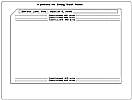Let us make a corner cut to reduce the possibility of the operator inverting or flipping the correct orientation of the "card" in the punch and reader.

The binary bit positions representing a binary "one" have had a small (2 millimeter?) hole arced through the paper card. This hole can be read by the machine by trying to spark through the hole or non-hole because paper has a higher dielectric strength than a paper with a hole.

The operator should be able to see the hole as the hole should transmit much more light than a non-hole.

Possible Pivot Comparator, non-existent

 To aid production speed and accuracy, I think we need an unmentioned operator aid. To aid the operator with the challenge of trying to compare the absolute magnitudes of the pivot coefficients described above, an unmentioned mechanical aid would seem useful. The operator would place the two Binary Data Cards for the next machine processing into two optical reader holders, and set the Comparator to focus on the line number (pivot coefficient) of the next ABC machine processing. These two lines of bit positions, some intact paper, some perforated by an arc, can be made visible to the operator adjacent to each other for easier viewing - using what ever smoke, mirrors and magnifications are necessary to cause this effect. Since the bit positions are likely to be 1/8 inches apart, vertically and horizontally, some back lighting and magnification is likely to be useful.
The method is explained in most high school algebra texts, and outlined below

Gaussian Forward Elimination

The game plan is determine the ratios of the two similarly positioned coefficients of two equations, such as the first column in

 ``` x + 3y - 2z - 1 = 0 2x + 5y - 2z - 6 = 0 ```
the ratio between the " x" and "2x" is 0.5 (by division).
multiply the second equation by 0.5, yielding
 ``` x + 3y - 2z - 1 = 0 x + 2.5y - z - 3 = 0 ```
depending on the signs of the coefficients, subtract or add the first equation to the second equation, yielding
 ``` (0x) - 0.5y + 2z - 4 = 0 ```
You can ignore the "(0x)"
replace the second equation with the result above,
and carefully continue on the same pattern to yield the
"Result of the Gaussian Forward Elimination" such as below

John Atanasoff ?invented? a scheme of doing the above operations using a rather simple machine,

 * without a formal multiply * the only division was by 2 (a sign extended right shift) * the controls to automatically eliminate an operator selected coefficient from two input equations.
The result was recorded, with electric sparks, onto an "Intermediate Result 'Card'"
to be used in further processing.

Gaussian Backward Substitution

Continuing on, using the one coefficient determined,
Back Substitute using standard algebra to form the

"Result of Gaussian Backward Substitution" below.

The exact recipe for

Gaussian Forward Elimination
Gaussian Backward Substitution
is best given in computer code, (easily found on the internet ;-))

 ``` Initial 4 equations, 4 unknowns +n.nnnnA +n.nnnnB +n.nnnnC +n.nnnnD = +n.nnnn +n.nnnnA +n.nnnnB +n.nnnnC +n.nnnnD = +n.nnnn +n.nnnnA +n.nnnnB +n.nnnnC +n.nnnnD = +n.nnnn +n.nnnnA +n.nnnnB +n.nnnnC +n.nnnnD = +n.nnnn Result of Gaussian Forward Elimination +n.nnnnA +n.nnnnB +n.nnnnC +n.nnnnD = +n.nnnn 0 +n.nnnnB +n.nnnnC +n.nnnnD = +n.nnnn 0 0 +n.nnnnC +n.nnnnD = +n.nnnn 0 0 0 +n.nnnnD = +n.nnnn Result of Gaussian Backward Substitution +n.nnnnA 0 0 0 = +n.nnnn 0 +n.nnnnB 0 0 = +n.nnnn 0 0 +n.nnnnC 0 = +n.nnnn 0 0 0 +n.nnnnD = +n.nnnn ```

(During the many operations to achieve the above results, the scaled 50 bit values could overflow. The operator is responsible for rescaling the two input equations, by dividing by 2 (shifting 1 bit right in the KA drum) and trying again.)

The operator then takes the result of the Gaussian Backward Substitution (above)
and using a desk calculator, obtains the desired unknowns

 ``` Result of desk calculator divisions A 0 0 0 = +n.nnnn 0 B 0 0 = +n.nnnn 0 0 C 0 = +n.nnnn 0 0 0 D = +n.nnnn ```
and gives these results to the ABC Customer Interface / Mathematician
who does some checking and submits these results to the customer :-))
 There are a number of enhancements to the original simple Gaussian Elimination. An internet search reveals these. They all depend upon the ability of the operator or supervisory control to compare the absolute values of the beginning and intermediate coefficient values to guide the selection of pivots to gain greater stability and accuracy. The above comparison mechanism is apparently not available to the ABC operator, so this simulation uses the original simple Gaussian Elimination.

If you have comments or suggestions, Send e-mail to Ed Thelen